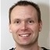0

# Accuracy of trigonometric functions

Theleo De Bruyn 4 months ago in IQANdesign updated 4 months ago

Hi there!

I have a question about the accuracy of triangulation calculation. A common math operator I use is "tan". I use this for calculating the slope [%] from the angle of inclination [°]. This has always worked fine for me but now I need a higher accuracy.

The Qcode I use in a math component:

Result:= tan(Angle)*100

Where:

Angle is in [°]

Result is in [%]

The result jumps from 0% to 0.17%. I would like to see an accuracy of 0.01%. Is there any other way to achieve this? Using sin/cos gave me the same results.

I have a Gyroscope Angle sensor with an accuracy of .01°. So the problem isn't the measuring.

Theleo De Bruyn+1

There is a limitation in the resolution on the trigonometric functions in IQANdesign, from the IQANdesign user manual:

Operand resolution for trigonometric operators is at least 0.1°. Result resolution is at least 3 decimals.

Depending on the application, you might be getting a more accurate result with other approximations, e.g.

Small-angle approximation - WikipediaThank you for the quick reply. I'll try a different approach using the suggested approximations.